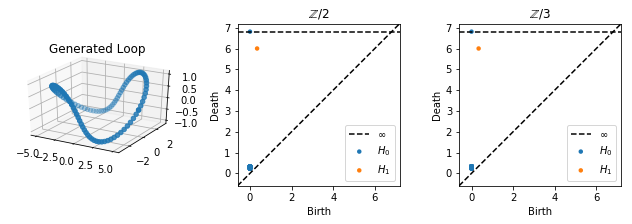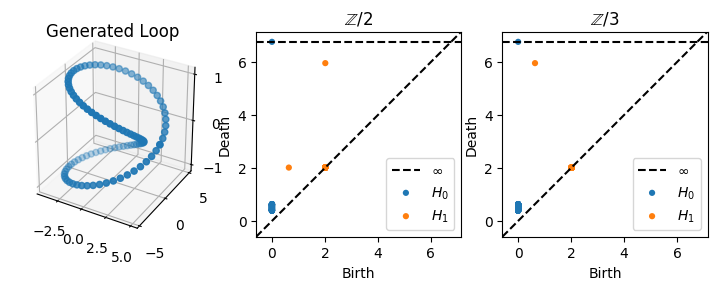# Moebius Strip And The Field of Coefficients¶

In this notebook we will explore an example in which the field of coefficients impacts the answer that 1 dimensional homology gives. This example demonstrates that contrary to common conventions which say to always use $$\mathbb{Z} / 2$$ (binary) coefficients there may be good reasons to use other fields, especially when there are twists.

First, we do all of the necessary imports as usual

:

import numpy as np
import matplotlib.pyplot as plt
from mpl_toolkits.mplot3d import Axes3D
from ripser import ripser
from persim import plot_diagrams


Now we will create a closed loop which lives on a 2-torus embedded in 3 dimensions. First, we will have the loop travel twice around the small circle (the “inner tube” part) for every one revolution around the large circle. Given a radius $$R$$ for the big loop and a radius $$r$$ for the small loop, we sample the following parametric curve

$$x(t) = (R + r \cos(2t)) \cos(t)$$

$$y(t) = (R + r \cos(2t)) \sin(t)$$

$$z(t) = r \sin(2t)$$

We then compute persistent homology using both $$\mathbb{Z} / 2$$ and $$\mathbb{Z} / 3$$ coefficients

:

## Step 1: Setup curve
N = 100 # Number of points to sample
R = 4   # Big radius of torus
r = 1   # Little radius of torus
X = np.zeros((N, 3))
t = np.linspace(0, 2*np.pi, N)
X[:, 0] = (R + r*np.cos(2*t))*np.cos(t)
X[:, 1] = (R + r*np.cos(2*t))*np.sin(t)
X[:, 2] = r*np.sin(2*t)

## Step 2: Compute persistent homology
dgms2 = ripser(X, coeff=2)['dgms']
dgms3 = ripser(X, coeff=3)['dgms']

fig = plt.figure(figsize=(9, 3))
ax.scatter(X[:, 0], X[:, 1], X[:, 2])
ax.set_aspect('equal')
plt.title("Generated Loop")
plt.subplot(132)
plot_diagrams(dgms2)
plt.title("$\mathbb{Z} / 2$")
plt.subplot(133)
plot_diagrams(dgms3)
plt.title("$\mathbb{Z} / 3$")

plt.tight_layout()
plt.show()Although the loop is very curved, we still see just one class forming in H1, and the persistence diagrams are the same for $$\mathbb{Z} / 2$$ and $$\mathbb{Z} / 3$$ coefficients; there is one class born at around $$0$$ which dies at $$r + R$$.

If, on the other hand, we have the loop go twice around the big circle for every once around the small circle; that is, the following parametric curve

$$x(t) = (R + r \cos(t)) \cos(2t)$$

$$y(t) = (R + r \cos(t)) \sin(2t)$$

$$z(t) = r \sin(t)$$

:

X[:, 0] = (R + r*np.cos(t))*np.cos(2*t)
X[:, 1] = (R + r*np.cos(t))*np.sin(2*t)
X[:, 2] = r*np.sin(t)

## Step 2: Compute persistent homology
dgms2 = ripser(X, coeff=2)['dgms']
dgms3 = ripser(X, coeff=3)['dgms']

fig = plt.figure(figsize=(9, 3))
ax.scatter(X[:, 0], X[:, 1], X[:, 2])
ax.set_aspect('equal')
plt.title("Generated Loop")
plt.subplot(132)
plot_diagrams(dgms2)
plt.title("$\mathbb{Z} / 2$")
plt.subplot(133)
plot_diagrams(dgms3)
plt.title("$\mathbb{Z} / 3$")

plt.show()In this case, we actually see a change in the diagrams for different field coefficients. For $$\mathbb{Z}/2$$, there is a class which is born around $$0$$ and which dies at $$r$$, and there is a class which is born at $$r$$ and dies at $$r + R$$. By contrast, for $$\mathbb{Z}/3$$, there is a single high persistence class which is born at around $$0$$ and which dies at $$r+R$$, just as before. In other words, using $$\mathbb{Z}/2$$ coefficients “splits” the bar from $$[0, r+R)$$ into two bars: $$[0, r)$$ and $$[r, r+R)$$.

Geometrically, what’s happening in this case is that the loop we have parameterized is the boundary of a Moebius strip, whose strip width is $$r$$. For $$\mathbb{Z}/2$$ coefficients, a class is born at $$0$$ but is bounded when the strip width fills in at $$r$$, at which point another class is born which is not topologically equivalent to the original class. By contrast, with $$\mathbb{Z} / 3$$ coefficients, the curve is not bounded when the strip is filled in at $$r$$; it is only bounded at the end when the entire torus fills in.